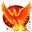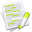#wx.lib.ogl.basic.PolygonShape¶

The PolygonShape class shape is defined by a number of points passed to the object’s constructor. It can be used to create new shapes such as diamonds and triangles.

##Class Hierarchy¶Inheritance diagram for class PolygonShape:

##Known Superclasses¶

wx.lib.ogl.basic.Shape

##Methods Summary¶

 __init__ Default class constructor AddPolygonPoint Add a control point after the given point. AttachmentIsValid Is attachment valid? CalculateBoundingBox Calculate the bounding box. CalculatePolygonCentre Recalculates the centre of the polygon, and ClearPoints Clear the points. Create Takes a list of wx.Points or tuples; each point is an offset DeletePolygonPoint Delete the given control point. GetAttachmentPosition Get attachment position. GetBoundingBoxMin Get minimum bounding box. GetNumberOfAttachments Get number of attachments. GetOriginalHeight Get the original height. GetOriginalPoints Get the original points. GetOriginalWidth Get the original width. GetPerimeterPoint Get the perimeter point. GetPoints Return the internal list of polygon vertices. HitTest Hit text MakeControlPoints Make control points. OnDraw The draw handler. OnDrawOutline The draw outline handler. OnSizingBeginDragLeft The sizing begin drag left handler. OnSizingDragLeft The sizing drag left handler. OnSizingEndDragLeft The sizing end drag left handler. ResetControlPoints Reset control points. Rotate Rotate about the given axis by the given amount in radians. SetOriginalHeight Set the original height. SetOriginalWidth Set the original width. SetSize Set the size UpdateOriginalPoints If we’ve changed the shape, must make the original points match the

##Class API¶

class PolygonShape(Shape)

The PolygonShape class shape is defined by a number of points passed to the object’s constructor. It can be used to create new shapes such as diamonds and triangles.

### Methods¶

__init__(self)

Default class constructor

Does not follow above statement, should it? or is Create called automagically?

AddPolygonPoint(self, pos)

Add a control point after the given point.

Parameters

pos – position of point

AttachmentIsValid(self, attachment)

Is attachment valid?

Parameters

attachment – ???

CalculateBoundingBox(self)

Calculate the bounding box.

CalculatePolygonCentre(self)

Recalculates the centre of the polygon, and readjusts the point offsets accordingly. Necessary since the centre of the polygon is expected to be the real centre of the bounding box.

ClearPoints(self)

Clear the points.

Create(self, the_points = None)

Takes a list of wx.Points or tuples; each point is an offset from the centre.

DeletePolygonPoint(self, pos)

Delete the given control point.

Parameters

pos – position of point

GetAttachmentPosition(self, attachment, nth = 0, no_arcs = 1, line = None)

Get attachment position.

Parameters
• attachment – the attachment ???

• nth – get nth attachment ???

• no_arcs – ???

• line – ???

GetBoundingBoxMin(self)

Get minimum bounding box.

GetNumberOfAttachments(self)

Get number of attachments.

GetOriginalHeight(self)

Get the original height.

GetOriginalPoints(self)

Get the original points.

GetOriginalWidth(self)

Get the original width.

GetPerimeterPoint(self, x1, y1, x2, y2)

Get the perimeter point.

Parameters
• x1 – the x1 position

• y1 – the y1 position

• x2 – the x2 position

• y2 – the y2 position

GetPoints(self)

Return the internal list of polygon vertices.

HitTest(self, x, y)

Hit text

Parameters
• x – the x position

• y – the y position

MakeControlPoints(self)

Make control points.

OnDraw(self, dc)

The draw handler.

OnDrawOutline(self, dc, x, y, w, h)

The draw outline handler.

OnSizingBeginDragLeft(self, pt, x, y, keys = 0, attachment = 0)

The sizing begin drag left handler.

OnSizingDragLeft(self, pt, draw, x, y, keys = 0, attachment = 0)

The sizing drag left handler.

OnSizingEndDragLeft(self, pt, x, y, keys = 0, attachment = 0)

The sizing end drag left handler.

ResetControlPoints(self)

Reset control points.

Rotate(self, x, y, theta)

Parameters
• x – the x position

• y – the y position

• theta – the theta

SetOriginalHeight(self, h)

Set the original height.

Parameters

w – the height

SetOriginalWidth(self, w)

Set the original width.

Parameters

w – the width

SetSize(self, new_width, new_height, recursive = True)

Set the size

Parameters
• new_width – the width

• new_height – the height

• recursive – not used

UpdateOriginalPoints(self)

If we’ve changed the shape, must make the original points match the working points with this function.